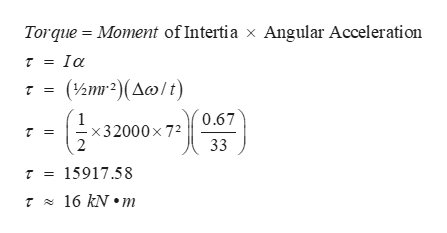# A merry-go-round accelerates from rest to 0.67 rad/s in 33s.Part A Assuming the merry-go-round is a uniform disk of radius 7.0 mand mass 3.20×104 kg, calculate the net torque required to accelerate it.r=

Question
393 views

A merry-go-round accelerates from rest to 0.67 rad/s in 33s.

Part A
Assuming the merry-go-round is a uniform disk of radius 7.0 m
and mass 3.20×104 kg, calculate the net torque required to accelerate it.

r=

check_circle

Step 1

Calulating the ...help_outlineImage TranscriptioncloseTorque Moment of Intertia x Angular Acceleration Ioa (2m2)(Acw/t) 0.67 - x32000 x 72 33 r = 15917.58 r16 kN m fullscreen

### Want to see the full answer?

See Solution

#### Want to see this answer and more?

Solutions are written by subject experts who are available 24/7. Questions are typically answered within 1 hour.*

See Solution
*Response times may vary by subject and question.
Tagged in

### Other